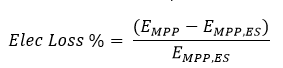# Electrical Shading Models and Calculation

## Fractional Effect –

This model assumes that all module strings in a table are strung together according to vertical rank. Below, an example portrays a PV table that consists of 2 strings each consisting of 8 modules.The table is partially shaded on the bottom corner, and due to the fact that at least one module in the bottom string gets shaded the entire string experiences power loss due to electrical shading. The fractional effect value determines how much electrical shading power loss will be applied. A fractional effect of 100% will have the entire strings power go to zero once a portion (of greater than 1%) gets shaded. This will determine the electrical beam shading factor that gets calculated for the DC field and, in turn, the effective irradiance that the modules will see (explained further in Shade Factor Calculation).

A 75% fractional effect will reduce the entire string’s electrical beam shading factor to 25% of the linear beam shading factor, essentially reducing the loss due to electrical shading by 25%.

The below figure defines certain variables used in the Shade Factor (UshBES) calculation. Xm and Ym are the module’s length and width dimension, respectively. The other two dimensions, Xs and Ys represent the distance, length and width respectively, that the shadow overlaps the module.Knowing these dimensions at each time step, PlantPredict is able to calculate the shading factor using the following equations:In which Z is the shading response table (examples of two such tables were provided in the c-SI 3 Diode Default and Custom Shading Table sections above). Once the shading factor has been determined, PlantPredict will then use that to compute the Global Effective Beam Irradiance (Ee,ES) after it has been adjusted for electrical shading.UshBES,filt is a modified version of the shading factor values (UshBES) that were calculated earlier, but filtered to convert any value that is to 1 (no shading applied) if the area of the module shaded is less than 1%.

The next tasks that the logic performs is to recalculate the cell temperature to reflect the fact that the module is being subjected to a new Global Effective Beam Irradiance value. This calculation is done based on the Module Temperature model that is selected in the simulation settings. To see this calculation please refer to the Module Temperature section lower in this document.

Once Ee,ES and Tcell,mod are determined, the last step is for PlantPredict to plug these into the functions that compute the 5 parameters of the I-V curve and subsequently the single diode model so that the maximum power point energy after electrical shading (EMPP,ES) can be found.

The Electrical Loss % (as seen in the results screen) is calculated as follows:In which EMPP is the maximum power point energy if it were to be calculated with linear shading.